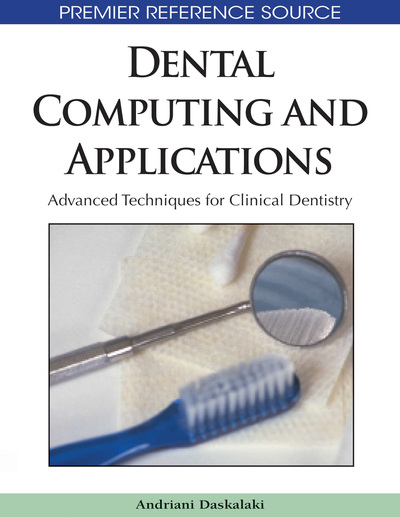# Finite Element Analysis and its Application in Dental Implant Research

Antonios Zampelis (School of Applied Mathematics and Physical Sciences, Greece) and George Tsamasphyros (School of Applied Mathematics and Physical Sciences, Greece)
DOI: 10.4018/978-1-60566-292-3.ch012
Available
\$33.75
List Price: \$37.50
10% Discount:-\$3.75
TOTAL SAVINGS: \$3.75

## Abstract

Biomechanical research has gained recognition in medical sciences. Osseointegrated dental implants, being medical devices functioning under constant load, are one of the focal points of such research. One of the most powerful tools for biomechanical research on dental implants is finite element analysis (FEA). This chapter will cope with basic elements of FEA research, the mechanical properties of bone and the various parts of dental implants, as well as delve into published literature on the subject.
Chapter Preview
Top

## Introduction

Finite element analysis (FEA) is a computer simulation technique used in engineering analysis. It uses a numerical technique called the finite element method (FEM). There are many finite element software packages available, both free and proprietary. The sophistication of this technique has rendered it an invaluable tool in biomechanical research.

The finite element analysis was first developed in 1943 by Richard Courant, who used the Ritz method of numerical analysis and minimization of variational calculus to obtain approximate solutions to vibration systems. Shortly thereafter, the work of M.J.Turner, R.W.Clough, H.C.Martin and L.J.Topp in 1956 established a broader definition of numerical analysis. The researchers centered on the “stiffness and deflection of complex structures”. Development of the finite element method in structural mechanics is usually based on an energy principle such as the virtual work principle or the minimum total potential energy principle.

In its applications, the object or system is represented by a geometrically similar model consisting of multiple, linked, simplified representations of discrete regions-i.e. finite elements on an unstructured grid. Equations of equilibrium, in conjunction with applicable physical considerations, such as compatibility and constitutive relations, are applied to each element and a system of simultaneous equations is constructed. The system of partial differential equations is solved for unknown values using the techniques of linear algebra or nonlinear numerical schemes, as appropriate.

In lay terms, the mathematical model is represented by a mesh geometrically identical to the object being studied. The mesh is broken down to elements. There is a set number of elements for a distinct mesh, hence the term finite element analysis. Each element is defined by points called nodes. Depending on the type of analysis, a wide variety of elements can be used, such as one-dimensional (straight or curved), two-dimensional (triangles or quadrilaterals), torus-shaped and three-dimensional (such as tetrahedrals and hexahedrals). As a force is applied, these interconnected elements start to move. Movement is defined by means of displacement of their nodes. This displacement is transformed, through the calculations, to stress and strain values (Figure 1). While being an approximate method, the accuracy of the FEA method can be improved by refining the mesh in the model using more elements and nodes.

Figure 1.

Close up of an implant cervix (right) and bone (left). The finite elements are depicted as the ares marked by white lines. Each quadrilateral element consists of four nodes. The different colors depict iso-stress areas

A common use of FEA is for the determination of stresses and displacements in mechanical objects and systems. However, it is also routinely used in the analysis of many other types of problems, including those in heat transfer, fluid dynamics and electromagnetism. FEA is able to handle complex systems that defy closed-form analytical solutions.

Finite element analysis is also frequently used in biological systems, for example in orthopedics, but also for dental implants. In fact, realization of the importance of biomechanical aspects in implant dentistry have rendered FEA an essential tool in dental implant research.

Among the subjects investigated in dental implantology are both material properties and biomechanical performance of implants and their components. The behavior of titanium parts, such as abutments and screws, has been put under scrutiny to determine their function under load and the possibility of failure due to fatigue. From a mechanical point of view, such studies are trivial.

On the other hand, the biological behavior of implants integrated in bone is a more complicated matter. A number of studies have investigated the effect of implant length, diameter, shape, as well as different placement configurations and the resulting stresses transmitted to the bone. Often, the effect of prosthetic material and shape of construction, such as the inclusion of cantilevers, is taken into consideration.

The main concern with the application of FEA in implant research is to which extent a mathematical model can represent a biological system. Published studies show a notable trend towards optimization of mathematical models. Improved software and a dramatic increase in easily available computational power have assisted in this trend.

## Complete Chapter List

Search this Book:
Reset